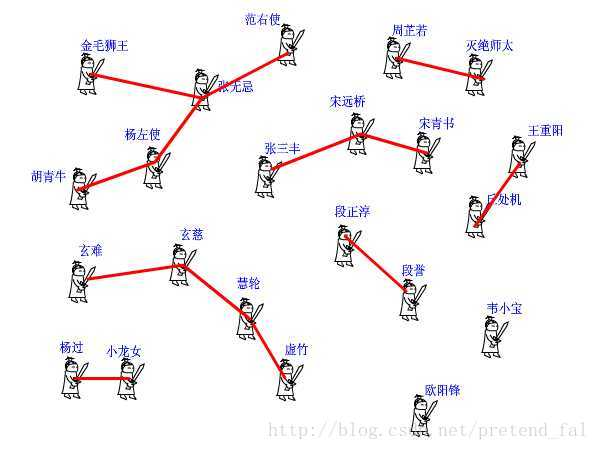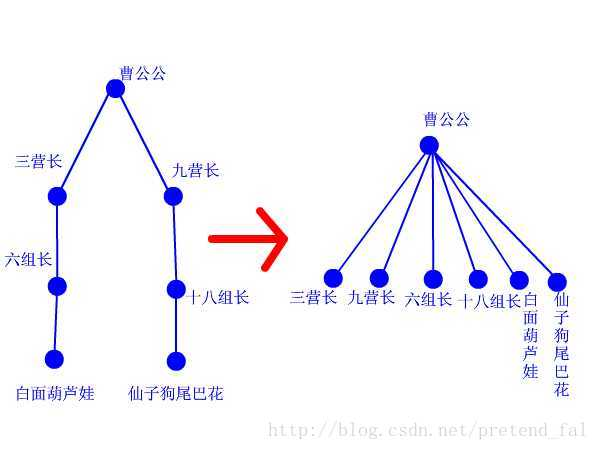Ⅰ 初始化：我们会把每一个点放入一个单独的集合，即 fa[x]=x，代表 x 所在的这个集合的代表元素是 x；

Ⅱ 查询：我们每一个集合的表现形式是一颗树，而所有的集合便表现为一个森林，所以我们递归地查询 x 所在的集合，一旦找到某一个点是一个集合的代表元素，那么我们就可以认为 x 与这个点在一个集合（而且一定在一个集合），并且这个集合是现在找到的这个点所代表的集合；

Ⅲ 合并： 假设我们希望把元素 A 与元素 B 合并到一个集合中，那么我们可以将这两个点所在的集合的代表元素合并到一个集合，至于这个过程，详情见代码；int find(int x)                         //查找我（x）的掌门
{
int r=x;                            //委托 r 去找掌门
while (pre[r]!=r)                   //如果 r 的上级不是 r 自己（也就是说找到的大侠他不是掌门 = =）
r=pre[r] ;                          // r 就接着找他的上级，直到找到掌门为止。
return  r ;                         //掌门驾到~~~
}


void join(int x,int y)                   //我想让虚竹和周芷若做朋友
{
int fx=find(x),fy=find(y);           //虚竹的老大是玄慈，芷若 MM 的老大是灭绝
if(fx!=fy)                           //玄慈和灭绝显然不是同一个人
pre[fx]=fy;                         //方丈只好委委屈屈地当了师太的手下啦
}


II find (R II x)
{
if(x==fa[x]) return x;
else return fa[x]=find(fa[x]);
//这个地方我们由 esle return find(fa[x]) 改为了
//return fa[x]=find(fa[x]);
//就是每次查询，我们就会把没有并在代表元素上的节点并到代表元素上；
//这样就可以使得树的深度较浅了；
}#include <bits/stdc++.h>
#define II int
#define IL inline
#define R register
#define I 123456
using namespace std;

IL void of(R II &a) {
R char c=getchar (); R II w=1, p=0;
while (c<'0'||c>'9') { if(c=='-') w=-1; c=getchar (); }
while (c>='0' && c<='9') { p=p*10+c-'0'; c=getchar (); }
a=w*p;
}

/* -------------------- Peipei -------------------- */

II fa[I];
II n,m;

II find (R II x)
{
if(x==fa[x]) return x;
else return fa[x]=find(fa[x]);
//这个地方我们由 esle return find(fa[x]) 改为了
//return fa[x]=find(fa[x]);
//就是每次查询，我们就会把没有并在代表元素上的节点并到代表元素上；
//这样就可以使得树的深度较浅了；
}

void join(R II x,R II y) { fa[x]=y; }

int main()
{
of(n); of(m);
for(R II i=1;i<=n;i++) fa[i]=i;
//初始化；
for(R II i=1,x,y,z;i<=m;i++)
{
of(x); of(y); of(z);
if(x==1) join(find(y),find(z));
//注意一定要传代表元素，保证正确性；
//不明白可以画图试一下；
else find(y)==find(z) ? printf("Y\n") : printf("N\n") ;
//查询是否在一个集合中；
}
return 0;
}


by pretend-fal;

END;

#### P`eipei

Why Be King When Can Be God.